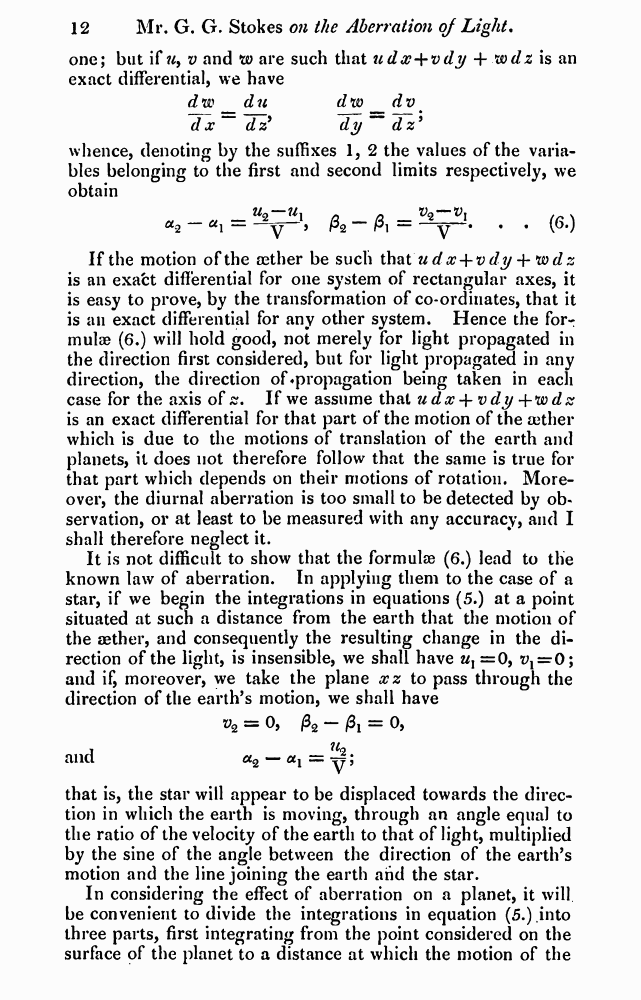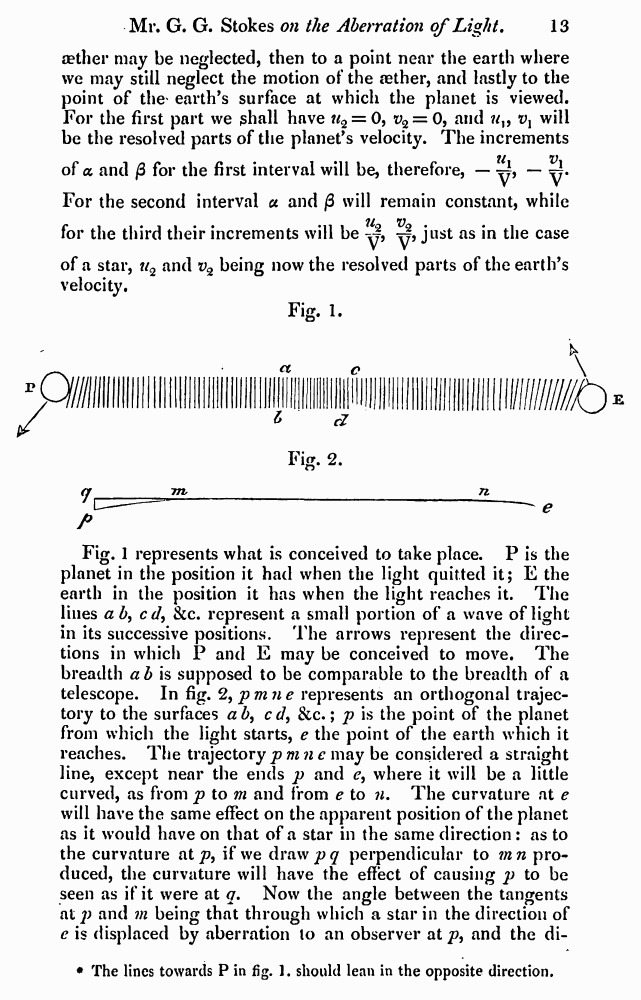﻿ G.G.Stokes "On the Aberration of Light" // Philosophical Magazine 1845, 27: Page 12

# G.G.Stokes "On the Aberration of Light" // Philosophical Magazine 1845, 27: Page 12

В начало   PDF, DjVu   <<<     Страница 12   >>>1  2  9  10  11  12 13  14  15 one; but if u> v and w are such that udx+vdy + wdz is an exact differentia], we have dw d u dw^dv d x~ dz9 dy~~dz9 whence, denoting by the suffixes 1, 2 the values of the variables belonging to the first and second limits respectively, we obtain = . . (6.) If the motion of the aether be such that udx + vdy -\-*wdz is an exafct differential for one system of rectangular axes, it is easy to prove, by the transformation of co-ordinates, that it is an exact differential for any other system. Hence the formulae (6.) will hold good, not merely for light propagated in the direction first considered, but for light propagated in any direction, the direction of ♦propagation being taken in each case for the axis of ss. If we assume that u dx + v dy + w dz is an exact differential for that part of the motion of the aether which is due to the motions of translation of the earth and planets, it does not therefore follow that the same is true for that part which depends on their motions of rotation. Moreover, the diurnal aberration is too small to be detected by observation, or at least to be measured with any accuracy, and I shall therefore neglect it. It is not difficult to show that the formulae (6.) lead to the known law of aberration. In applying them to the case of a star, if we begin the integrations in equations (5.) at a point situated at such a distance from the earth that the motion of the aether, and consequently the resulting change in the direction of the light, is insensible, we shall have ux =0, ^=0; and if, moreover, we take the plane xz to pass through the direction of the earth’s motion, we shall have = 0, /32 = 0, and ct2 — &i = —; that is, the star will appear to be displaced towards the direction in which the earth is moving, through an angle equal to the ratio of the velocity of the earth to that of light, multiplied by the sine of the angle between the direction of the earth’s motion and the line joining the earth and the star. In considering the effect of aberration on a planet, it will be convenient to divide the integrations in equation (5.) into three parts, first integrating from the point considered on the surface of the planet to a distance at which the motion of the aether may be neglected, then to a point near the earth where we may still neglect the motion of the aether, and lastly to the point of the' earth’s surface at which the planet is viewed. For the first part we ghall have u% = 0, t>2 = 0, and vx will be the resolved parts of the planet’s velocity. The increments tt V of a and /3 for the first interval will be, therefore, — y, — ^. For the second interval a and /3 will remain constant, while 11 v for the third their increments will be y, y, just as in the case of a star, w2 and i>2 being now the resolved parts of the earth’s velocity. Fig. 1. P Fig. 1 represents what is conceived to take place. P is the planet in the position it had when the light quitted it; E the earth in the position it has when the light reaches it. The lines a b, cd, &c. represent a small portion of a wave of light in its successive positions. The arrows represent the directions in which P and E may be conceived to move. The breadth a b is supposed to be comparable to the breadth of a telescope. In fig. %pmne represents an orthogonal trajectory to the surfaces a b, cd, &c.; p is the point of the planet from which the light starts, e the point of the earth which it reaches. The trajectorypmnc may be considered a straight line, except near the ends p and e, where it will be a little curved, as from p to m and from e to n. The curvature at e will have the same effect on the apparent position of the planet as it would have on that of a star in the same direction : as to the curvature at p, if we draw jpy perpendicular to mn produced, the curvature will have the effect of causing p to be seen as if it were at q. Now the angle between the tangents at p and m being that through which a star in the direction of e is displaced by aberration to an observer at p, and the di- The lines towards P in fig. 1. should lean in the opposite direction.